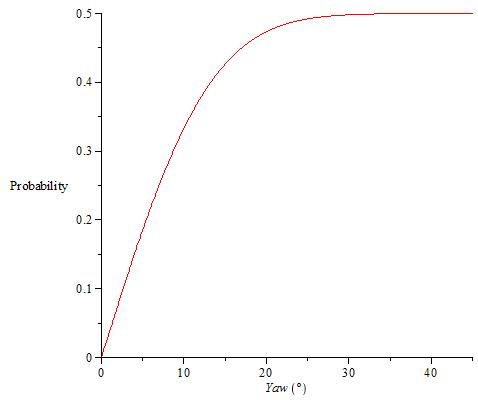## Monday, September 3, 2012

The subject of yaw is of vital importance when trying to design aerodynamic components. Typical values of yaw seen during cycling are intimately relationed with the speed of the cyclist and, therefore, the target client of these components

Dan Connelly developed some time ago a probability function for yaw motivated by some conflicts between ZIPP and HED about typical yaw values. This probability function used a Maxwellian distribution to describe wind speed and assumed that all the headings and wind directions were equally probable. The relation between wind speed at the height of a meteorological station and at bike level was obtained using the Hellman formula. I haven't checked all the process to obtain this function but Dan is a clever guy so I don't expect any mistakes to be found. My only concern was the calculation of v0, the standard deviation of the velocity components of the Maxwellian distribution. Dan assumed, maybe using a conclusion that is valid for normal distributions, that the peak of the probability function was equal to the average value of the probability function for speeds. Hellman formula works for average values of wind speeds so a correct relation between v0 and the average wind speed should be used. For this Maxwellian distribution for speeds, the average is given by the following limit:
Working it, we get:
Now we can calculate v0 as a function of the average wind speed at bike level that is obtained using the Hellman formula. The following two graphics show the influence of this modification in the wind speed and yaw probability functions that Dan showed.

As you can see, considering that the average wind speed is equal to v0, there is an underestimation of the presence of low wind speeds and yaw values.

Mavic showed a probability function of yaw determined through experimental studies during the development of the new CXR80 wheel. There isn't many details about how that function was obtained (wind speeds, bike speeds, etc) but I have tried to replicate it using the yaw probabilty function that Dan developed. First, I fitted a normal distribution to Mavic's plot. The plot misleads a little bit because it shows the CDF between every tick - 1.25º and + 1.25º but I have calculated the standard deviation using the CDF of an arbitrary division of the x scale. The normal distribution and the CDF of yaw obtained from Mavic's data are the following ones:Explanation: If you draw a vertical line taking as origin any abscise (let's call it Y), the ordinate of the intersection between that line and the function multiplied by 200 represents the percentage of time that you ride in the interval [-Y,Y]

That's all for today. Greetings